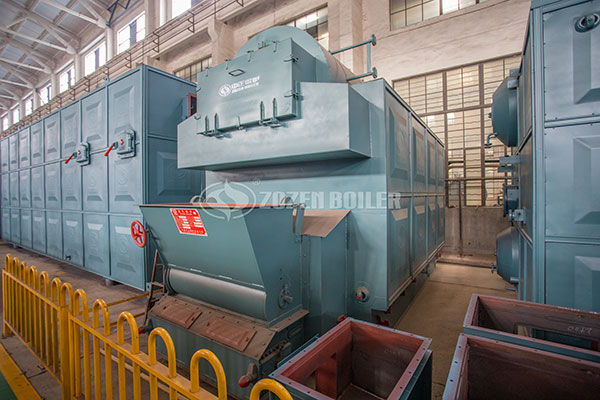# The Operating Cost of a Biomass Fired Pellet Boiler?

January 22, 2020 Tags:

Fuel cost is related to the competitiveness of an enterprise and whether it can adapt to the increase in costs after coal is banned. Peanut shell briquette fuel is a new type of environmentally friendly fuel. It mainly uses the fruit shell of new agricultural peanuts. Before processing into peanut shell briquettes, peanut shells have been a waste. This environmentally friendly fuel is used in a large number of industrial boilers and winter heating, so how much is the operating cost of peanut shell briquette fuel?Let’s take the heating of 10 tons of water to 45 degrees as an example. Let us calculate the cost of peanut shell briquettes, coal, gas and other energy sources. According to industry knowledge, the heat required to raise the temperature of 1 kg of water by 1 degree is 1 kcal. Let’s take 5 ° C to 45 ° C as an example. The calculation method is as follows:

The heat required to heat 1 kg of water at 5 degrees to 45 degrees is: (45-5) × 1 kcal = 40 kcal, 10 tons of water is 10,000 kg, and 10 tons of water to 45 degrees is 10000 × 40. = 400,000 kcal.

1.  If coal is used as fuel, 10 tons of water will be heated to 45 degrees.

The calorific value of coal is generally calculated at 5000 kcal / kg, the thermal efficiency of coal-fired boilers is calculated at 65%, and the unit price of coal is calculated at an average price of 0.6 yuan / kg: 400,000 kcal ÷ 5000 kcal. 70% utilization rate to calculate: 48 ÷ 0.7 = 68 yuan. Conclusion The coal cost for heating 10 tons of water to 45 degrees is 68 yuan.

2. If the peanut shell briquette is used as fuel, the running cost of peanut shell briquette fuel required for heating 10 tons of water to 45 degrees

The calorific value of peanut shell briquette fuel is generally 3600-3800 kcal, and the lowest 3600 kcal / kg is calculated. The thermal efficiency of peanut shell briquette fuel biomass boiler is calculated at 80%. Calculated at an average price of 0.5 yuan / kg: 400,000 kcal ÷ 3600 kcal X 0.5 = 55.6, calculated based on 80% utilization rate: 55.6 ÷ 0.8 = 69.5 元 Conclusion The cost of peanut shell briquette fuel to heat 10 tons of water to 45 degrees is 69.5 yuan.

3. If gas is used as fuel, the operating cost of gas required to heat 10 tons of water to 45 degrees

The calorific value of natural gas is generally calculated at 7500 kcal / cubic, the thermal efficiency of gas boilers is calculated at 95%, and the unit price of gas is calculated at an average price of 3.2 yuan / cubic: 400,000 kcal ÷ 7500 kcal. X 3.2 yuan = 170.6 yuan, 95 Calculate the utilization ratio of%: 55.6 ÷ 0.95 = 179.5 yuan. Conclusion The cost of natural gas for heating 10 tons of water to 45 degrees is 179.5 yuan.Based on the above simple calculations, it can be seen that a comprehensive measurement of the operating costs of various boilers, coal, peanut shell briquette fuel, and gas boilers is as follows: coal <peanut shell briquette fuel <gas, coal, and peanut shell The cost of briquettes is not much different, the cost of gas is more than twice that of coal and peanut shell briquette fuel. Therefore, from a cost perspective, peanut shell briquette fuel and coal costs are dominant, but coal pollution is not allowed. Gas is environmentally friendly, but gas costs are higher. If conditions permit, peanut shell briquettes are used instead of coal.